/

### Function Description

Returns the (Pearson) correlation coefficient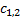between two series,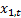and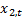.

The correlation coefficient is calculated as: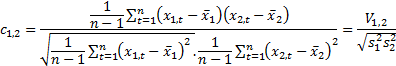where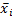and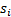are the mean and (sample) standard deviations of the-th series etc. and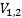is the (sample) covariance between the two series.

Contents | Prev | Next